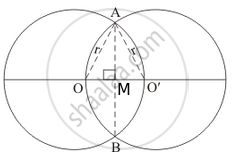Advertisement Remove all ads

# Two equal circles of radius r intersect such that each passes through the centre of the other. The length of the common chord of the circle is - Mathematics

MCQ

Two equal circles of radius r intersect such that each passes through the centre of the other. The length of the common chord of the circle is

#### Options

• $\sqrt{r}$

• $\sqrt{2}r AB$

• $\sqrt{3}r$

• $\frac{\sqrt{3}}{2}$

Advertisement Remove all ads

#### Solution

$\sqrt{3}r$

We are given two circles of equal radius intersect each other such that each passes through the centre of the other.

We need to find the common chord.

We have the corresponding figure as follows:AO AO = r (radius)

And OO = r

SoΔOAO is an equilateral triangle.

We know that the attitude of an equilateral triangle with side r is given by (sqrt{3})/2

That is AM =   (sqrt{3})/2r

We know that the line joining centre of the circles divides the common chord into two equal parts.

So we have

AB = 2AM

= 2.(sqrt(3))/2r

AB = sqrt(3)r

Is there an error in this question or solution?
Advertisement Remove all ads

#### APPEARS IN

RD Sharma Mathematics for Class 9
Chapter 15 Circles
Q 26 | Page 112
Advertisement Remove all ads

#### Video TutorialsVIEW ALL 

Advertisement Remove all ads
Share
Notifications

View all notifications

Forgot password?
Course